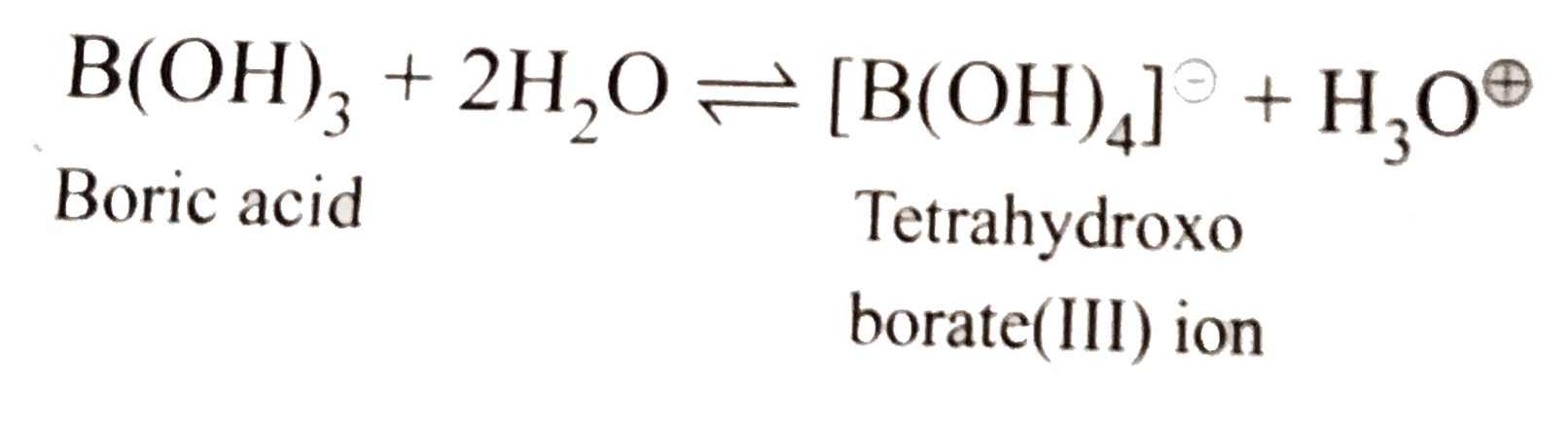# What happens when a. Borax is heated strongly. b. Boric acid is added to water. c. Aluminium is treated with dil NaOH. d. BF_(3) is reacted with a

76 views

closed
What happens when
a. Borax is heated strongly.
b. Boric acid is added to water.
c. Aluminium is treated with dil NaOH.
d. BF_(3) is reacted with ammonia.

by (69.2k points)
selected by

a.When borax is strongly heated, a transparent glassy bead which consists of sodium metaborate and boric anhydride is formed.
underset("Borax")(Na_(2)B_(4)O_(7).10H_(2)O)overset(Delta)toNa_(2)B_(4)O_(7)+10H_(2)O
Na_(2)B_(4)O_(7)underset("Red Heat")overset(Delta)tounderset(ubrace({:(" Sodium, Boric"),("metaborate, anhydride"):})_("Transport glassy bead"))(2NaBO_(2)" + "B_(2)O_(3))
b. Boric acid behaves as a Lewis acid and hence accepts overset(ө)OH ion form H_(2)O and realeses H^o+ in the solution.
c. 2Al_((s))+underset(("dil"))(NaOH_((aq)))+6H_(2)Oto2Na^(o+)[Al(OH)_(4)]_((aq))^()+3H_(2(g)) or underset("Sodium meta-aluminate(III)")(Na_(2)AlO_(2).2H_(2)O)
d.F_(3)B+:NH_(3)to[F_(3)Blarr:NH_(3)]
BF_(3) is a Lewis acid and accepts a pair of electron from N of NH_(3) molecule to form an adduct,
[F_(3)Blarr:NH_(3)]